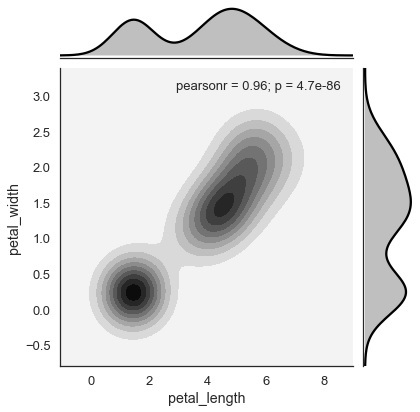# Seaborn - Kernel Density Estimates

#### Data Visualization using MatPlotLib & Seaborn

11 Lectures 4 hours

#### Seaborn with Python

11 Lectures 2.5 hours

#### Data Visualization in Python Using Seaborn Library

20 Lectures 2 hours

Kernel Density Estimation (KDE) is a way to estimate the probability density function of a continuous random variable. It is used for non-parametric analysis.

Setting the hist flag to False in distplot will yield the kernel density estimation plot.

## Example

```import pandas as pd
import seaborn as sb
from matplotlib import pyplot as plt
sb.distplot(df['petal_length'],hist=False)
plt.show()
```

## Output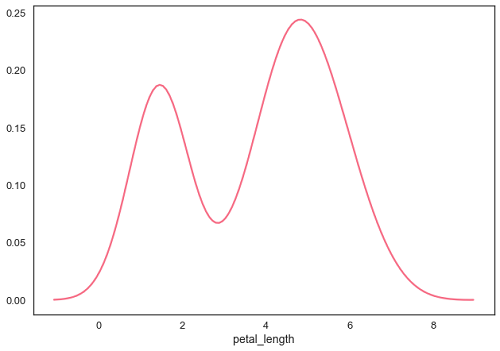## Fitting Parametric Distribution

distplot() is used to visualize the parametric distribution of a dataset.

### Example

```import pandas as pd
import seaborn as sb
from matplotlib import pyplot as plt
sb.distplot(df['petal_length'])
plt.show()
```

### Output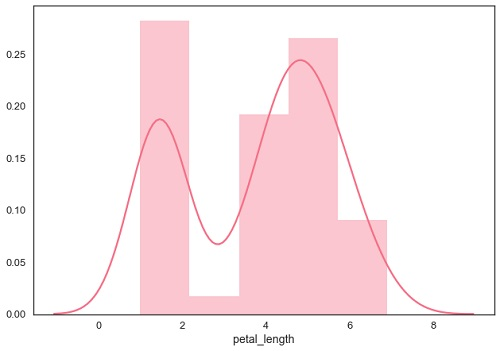## Plotting Bivariate Distribution

Bivariate Distribution is used to determine the relation between two variables. This mainly deals with relationship between two variables and how one variable is behaving with respect to the other.

The best way to analyze Bivariate Distribution in seaborn is by using the jointplot() function.

Jointplot creates a multi-panel figure that projects the bivariate relationship between two variables and also the univariate distribution of each variable on separate axes.

## Scatter Plot

Scatter plot is the most convenient way to visualize the distribution where each observation is represented in two-dimensional plot via x and y axis.

### Example

```import pandas as pd
import seaborn as sb
from matplotlib import pyplot as plt
sb.jointplot(x = 'petal_length',y = 'petal_width',data = df)
plt.show()
```

### Output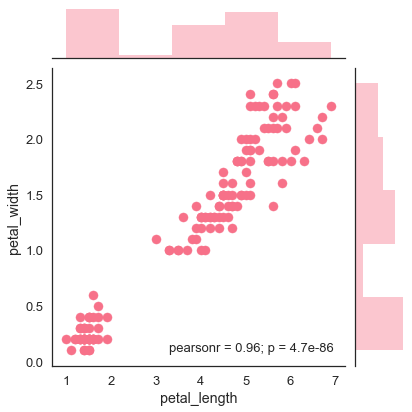The above figure shows the relationship between the petal_length and petal_width in the Iris data. A trend in the plot says that positive correlation exists between the variables under study.

### Hexbin Plot

Hexagonal binning is used in bivariate data analysis when the data is sparse in density i.e., when the data is very scattered and difficult to analyze through scatterplots.

An addition parameter called ‘kind’ and value ‘hex’ plots the hexbin plot.

### Example

```import pandas as pd
import seaborn as sb
from matplotlib import pyplot as plt
sb.jointplot(x = 'petal_length',y = 'petal_width',data = df,kind = 'hex')
plt.show()
```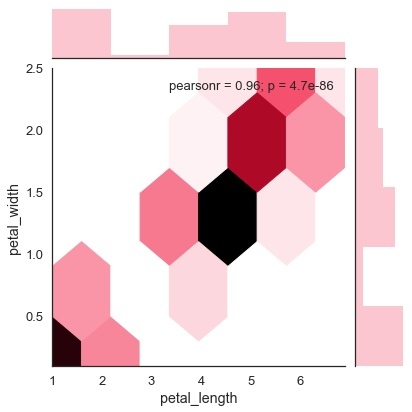## Kernel Density Estimation

Kernel density estimation is a non-parametric way to estimate the distribution of a variable. In seaborn, we can plot a kde using jointplot().

Pass value ‘kde’ to the parameter kind to plot kernel plot.

### Example

```import pandas as pd
import seaborn as sb
from matplotlib import pyplot as plt